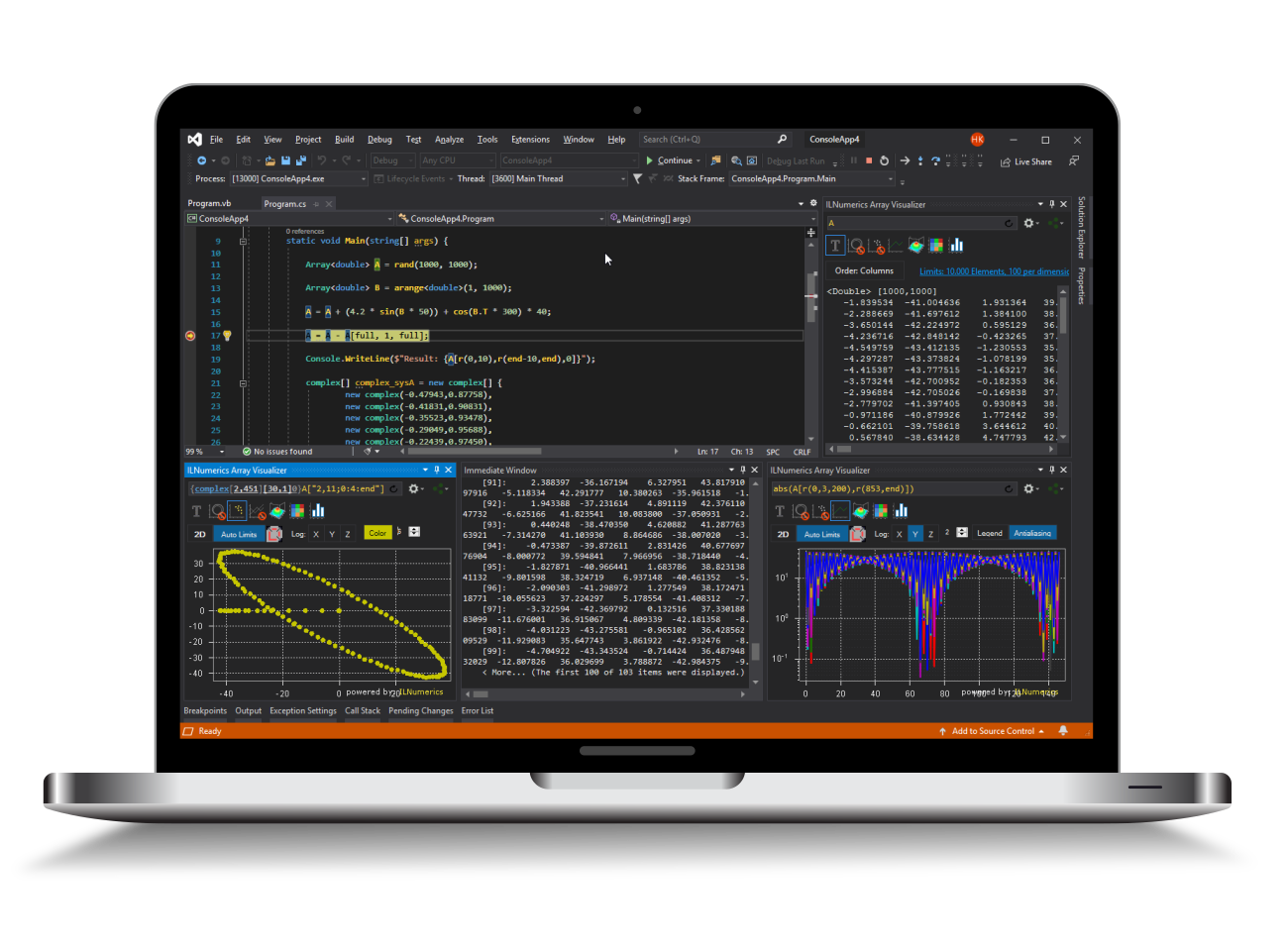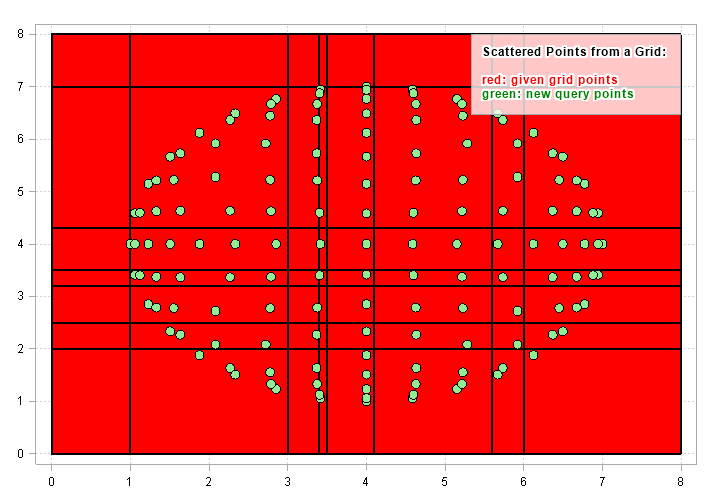Industrial Data Science
in C# and .NET:
Simple. Fast. Reliable.ILNumerics - Technical Computing

Modern High Performance Tools for Technical

Computing and Visualization in Industry and Science

tgt

# Scattered Data Interpolation from Grids

ILNumerics Interpolation Toolbox supports the interpolation of scattered data. In contrast to general scattered interpolation, this section deals with the special case of scattered query points interpolation while the known data points are ordered as a rectilinear grid.Talking about grids implies that the data are measured in $R^2\dots R^n$. One dimensional data are handled in a seperate article.

## Overview

For this kind of interpolation the source data grid, again, must be rectilinear but is not necessarily required to be regular, i.e.: the spacing between adjacent grid elements may vary within each dimension.

One example of a rectilinear grid (red) with scattered data (green) is given in the following image:Here, the known source samples were acquired at the grid crossings of the red areas. All tiles formed by the grid lines are rectangles: they show all angles of 90° only. However, these rectangles are not necessarily congruent, as can be clearly seen from the image above.

Regular grids with equal spacings are a special case of the general non-regular grids and applicable for the methods in this section similarly.

The green dots from the image above do not show a gridded order. They are distributed arbitrarily over the definition range. In your application, there will be some reasoning about individual query positions, though. However, such structure would not be taken into account by any of the interpolation methods described in the remainder of this section.

## Functions for Scattered Data Interpolation on Grids

The following functions allow the interpolation of scattered points on gridded data. They are all found in the ILNumerics Interpolation Toolbox as public static members of the ILNumerics.Toolboxes.Interpolation class. You will notice the similarity to the corresponding methods for gridded query points interpolation: the methods share the same name, suffixing an 's' only:

Function Description Dimension
interp2s() All interpolation methods on 2-dimensional data. Dimension order is expected in meshgrid format (rows first).
interp3s() All interpolation methods on 3-dimensional data. Dimension order is expected in meshgrid format (rows first).
interpns() All interpolation methods on n-dimensional data. Dimension order is expected in natural format (columns first).
Specialized interpolation methods
splinens() Spline interpolation methods on n-dimensional data. See here. Dimension order is expected in natural format (columns first).

All functions are specifically designed for multidimensional data. General interpolation is performed by the interp2s, interp3s, and interpns interface. This gives access to all interpolation methods on know, gridded points: linear, nearest-/next-/previous neighbor, polynomial, piecewise cubic and spline interpolation. For multidimensional spline interpolation with non-standard coundary conditions a specialized interface splinens() is provided and described in the article on spline interpolation.

In the following paragraphs the interp?s() functions are described.

## Interpolation on 2-dimensional Grids: interp2s()

The interp2s() function creates a set of scattered, newly interpolated points from a 2D grid of known values. The grid of the known values V is arbitrarily defined by the parameters X1 and X2. The function allows the definition of the interpolation method and, optionally, of the extrapolation behavior.

Example: Interpolate and extrapolate a function along an arbitrary line on a grid of known values.

The interpolation is performed in the line:

The following data are given to the interp2() function:

• V - the matrix of data values,
• X1 and X2 - define the grid positions (coordinates) of the known points,
• Xn1 and Xn2 - define the coordinates of the query points to be interpolated, and
• method - the interpolation method.

### Definition of known Points

The matrix V in the example above stores the values of the known points. The positions for the known points are defined by the X1 and X2 variables. Since the points are expected to be ordered as a grid, it is sufficient to define the coordinates of the vertical and horizontal grid lines as vectors of positions in X1 and X2. Those vectors must contain strictly monotonically increasing elements.

Alternatively, X1 and X2 can be matrices of the same size as V. In this case, the value, as well as the x and y coordinates of a known point are found at matching elements in all matrices: V, X1 and X2.

As a third alternative, X1 or X2 can be provided as null which will lead to the auto-generation of the position vectors: starting at 0, step size 1.0 over the length of the corresponding dimension in V.

### Order of Dimensions

An important distinction is made for the order of the dimensions in V: interp2s() and interp3s() (just as their counterparts interp2() and interp3()) are optimized for plotting purposes. I.e.: they expect the first dimension to go along the rows, the second dimension to go along the columns of V. This corresponds to the common look and feel of most popular plots: the x axis runs horizontally while the y axis goes vertically. In ILNumerics and many other similar systems, this order is associated with the output of the meshgrid() function:

Note, that the vector of grid points given as X parameter is reflected in the output along the rows. Similarly, the Y values starting at -10 go along the columns as can be seen from the 2nd output of meshgrid:

Keep in mind that it is only interp2s() and interp3s() which expose this ordering of dimensions. interpns() and any other N-D functions expects the dimensions of V in their 'natural' format, i.e.: the first dimension runs along the columns, the second dimension is associated with the rows.

### Definition of new Query Points

The set of newly interpolated points is defined by the arrays Xn1 and Xn2. Since these points are not expected to expose an ordered structure, Xn1 and Xn2 define the coordinates of the scattered points directly.

• Xn1 and Xn2 are vectors of the same length or arrays with the same number of elements.
• Xn1 defines the coordinates for the first dimension ('x axis'), Xn2 the coordinates for the second dimension ('y axis').
• Each entry in Xn1 and Xn2 corresponds to exactly one new query point.
• Points with coordinates outside of the definition range are allowed and will be considered as 'out-of-range' values (see below).
• The result returned from interp2s() will always be a vector with length corresponding to the number of query points defined in Xn1 and Xn2.

### Optional Parameters

Since the definition of new query points is obligatory in the case of scattered data, most of the parameters are obligatory and cannot be omitted. Refer to the apidoc for interp2s for a detailed description of all parameters.

### Extrapolation, Out-of-Range Values

By default, interp2s() assigns NaN to any new query point laying outside of the range defined for V. This behavior can be controlled by utilizing the overload of interp2s() which provides the outOfRangeValues parameter. Here come the options:

• Set this value to a numerical constant or NaN. This constant will be assigned to all out-of-range values. Note that setting this value to NaN leads to the same result than the utilization of the interp2s() overload without the outOfRangeValues parameter.
• Alternatively, if null is given for the outOfRangeValues parameter, values for out-of-range query points are computed by extrapolation from known query points using the selected interpolation method.

Example: Linear interpolation of a scattered line on a 2D grid.

The data set V is simply defined as follows:

Now we interpolate 13 points along an imaginary line running vertically, straight through the center of the matrix, parallel to the 2nd and the 3rd column. We do not specify actual values for the X1 nor the X2 parameter. Instead, the positions to the values in V are auto-defined as laying on a regular grid of stepsize 1, starting at 0. The X-coordinate of our scattered points is 1.5, Y coordinates vary from -1 ... 5:

We have defined several points outside the definition range of V. These points are set to NaN by default. If we want interp2() to extrapolate instead, we use the 2nd overload of interp2() and set the outOfRangeValues parameter to null:

## Interpolation of 3-dimensional Grids: interp3s()

In the 3-dimensional case the interp3s() function can be used in a very similar way. The function exposes a third parameter for defining the grid positions X3 of the known values and the parameter Xn3 for the new query point positions. The length of Xn3 must match the length of Xn1 and Xn2. All other parameters and options are applicable similarly.

## Interpolation of n-dimensional Grids: interpns()

In the general case of n-dimensional data V the interface of Interpolation.interpns() must allow the definition of arbitrary numbers of dimensions. Therefore, X-arrays are wrapped into a cell array, allowing to provide multiple grid definitions at once. However, the general scheme of providing grid position data X for the known values V stay the same.

The definition of new query points for interpolation in N-dimensions is done by providing a single matrix Xn to the interpns() function. As by convention in ILNumerics, points defined in Xn are stored in the columns of the matrix.

Example: 3-dimensional cubic interpolation and extrapolation of scattered points on a grid.

Keep the following rules in mind:

• The dimensionality of the problem is determined by the number of dimensions in V.
• The order of dimensions for interpns() differs from interp2s() and interp3s(): the first dimension is associated with the column in V, the second dimension with the rows. Higher dimensions are layed out as expected.
• X can define the grid positions for any dimension in V. Any dimension not defined is handled with the same default rules as for interp2s.
• All positional parameters X and Xn are obligatory.
• All interpolation methods from the enum InterpolationMethod are applicable.

Refer to the apidoc class reference documentation for interpn() for more details.

## A Note on Performance

All interpolation methods in this section (as so for all in ILNumerics Interpolation Toolbox) are carefully hand tuned for best performance and smallest resource consumption. They parallelize on multicore systems and are well prepared to be used on large data sets and in low latency scenarios.

See also: